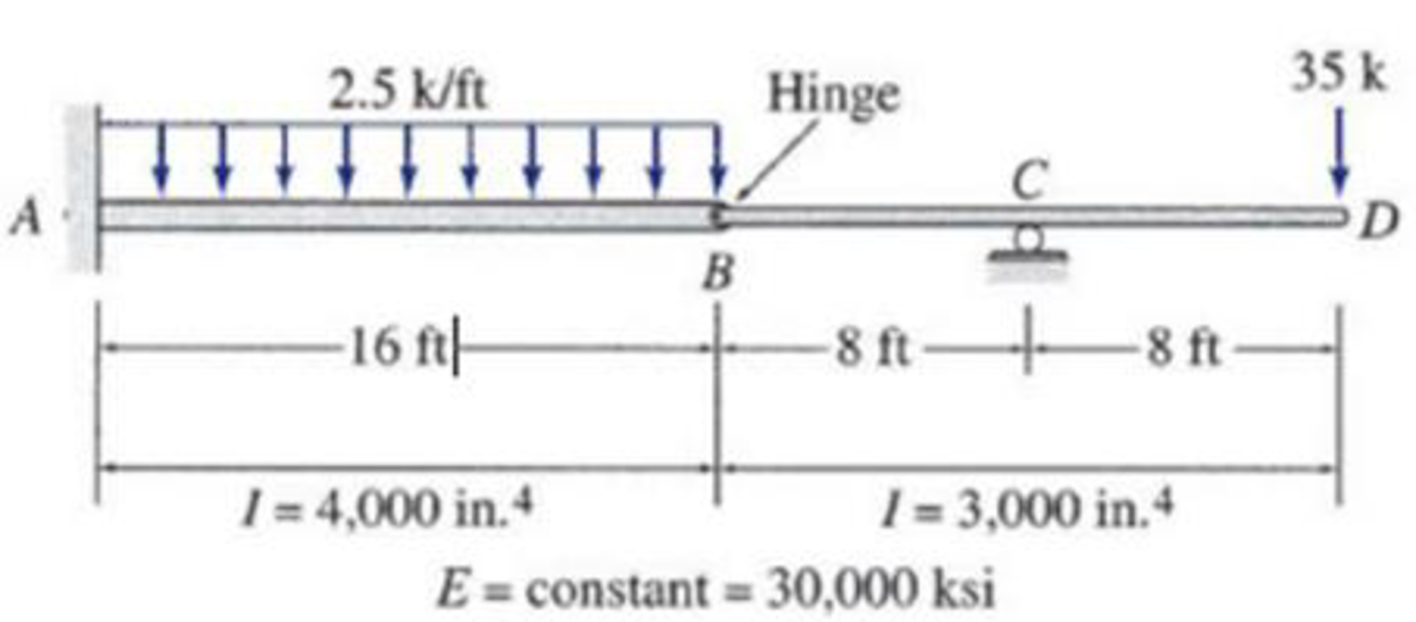# 6.59 and 6.60 Use the conjugate-beam method to determine the slopes and deflections at points at points B and D of the beams shown in Figs. P6.33 and P6.34. FIG. P6.34, P6.60

#### Solutions

Chapter
Section
Chapter 6, Problem 60P
Textbook Problem
9 views

## 6.59 and 6.60 Use the conjugate-beam method to determine the slopes and deflections at points at points B and D of the beams shown in Figs. P6.33 and P6.34.FIG. P6.34, P6.60

To determine

Find the slope θB&θD and deflection ΔB&ΔD at point B and D of the given beam using the moment area method.

### Explanation of Solution

Given information:

The Young’s modulus (E) is 30,000 ksi.

The moment of inertia of the section AB is (I) is 4,000in.4.

The moment of inertia of the section BD is (I) is 3,000in.4.

Calculation:

Consider flexural rigidity EI of the beam is constant.

To draw a M/EI diagram, the reactions are calculated by considering all the loads acting in the beam. The calculation of positive moment at point A is calculated by simply considering the point load of 35 kips and the reactions calculated by considering all the loads acting in the beam.

Show the free body diagram of the given beam as in Figure (1).

Refer Figure (1),

Consider upward is positive and downward is negative.

Consider clockwise is negative and counterclowise is positive.

Refer Figure (1),

Consider reaction at A and C as RA and RC.

Determine the reaction at D;

RC×(8)(35×16)=0RC=5608RC=70kips

Determine the reaction at support A;

V=0RA+RC(2.5×16)35=0RA=7570RA=5kips

Determine the moment at A:

MA=(35×32)+(70×24)(25×16×162)=240kips-ft=240kips-ft(Clockwise)

Show the reaction of the given beam as in Figure (2).

Determine the bending moment at B;

MB=70×835×16=560560=0

Determine the bending moment at C;

MC=(35×8)=280kips-ft

Determine the bending moment at D;

MD=(5×32)(70×8)240+(2.5×16×(162+16))=720+240+960=0

Determine the positive bending moment at A using the relation;

MA=(35×32)+70×24=1,120+1,680=560kips-ft

Show the reaction and point load of the beam as in Figure (3).

Determine the value of M/EI;

MEI=560kips-ftEI

Substitute 4I3 for I.

MEI=560kips-ftE(4I3)=1,680kips-ft4EI=420kips-ftEI

Show the M/EI diagram of the beam as in Figure (4).

Show the conjugate beam as in Figure (5).

Determine the support reaction at support B;

RB×8+1EI[13×240×16×(8+34×16)12×16×420×(8+23×16)+12×8×280×(13×8)]=0RB=25,60062,731+2,9878EIRB=4,268kips-ft2EIRB=4,268kips-ft2EI()

Determine the shear force at B (left) using the relation;

SB(left)=[12×b1×h113×b2×h2]

Substitute 16 ft for b1, 420EI for h1, 16 ft for b2, and 240EI for h2.

SB(left)=[12×16×420EI13×16×240EI]=2,080kips-ft2EI

Determine the slope at B (left) using the relation;

SB(left)=2,080kips-ft2EI

Substitute 30,000 ksi for E and 4,000in.4 for I.

Hence, the slope at B (left) is 0.0033rad_.

Determine the slope at B (right) using the relation;

θB(right)=θB(left)RB

Substitute 2,080kips-ft2EI for θB(left) and 4,268kips-ft2EI for RB.

θB(right)=2,080kips-ft2EI4,268kips-ft2EI=2,188kips-ft2EI

Substitute 30,000 ksi for E and 4,000in.4 for I.

θB(right)=2,188kips-ft2×(12in.1ft)230,000×4,000=0

### Still sussing out bartleby?

Check out a sample textbook solution.

See a sample solution

#### The Solution to Your Study Problems

Bartleby provides explanations to thousands of textbook problems written by our experts, many with advanced degrees!

Get Started

Find more solutions based on key concepts
Select an HP-shape for the conditions of Problem 4.6-3. Use Fy=50 ksi. a. Use LRFD. b. Use ASD.

Steel Design (Activate Learning with these NEW titles from Engineering!)

In a commercial water heater, 20 gallons/min of water is heated from 60 F to 140 F. Calculate the amount of ene...

Engineering Fundamentals: An Introduction to Engineering (MindTap Course List)

Write the SQL code that will save the changes made to the EMP_1 table.

Database Systems: Design, Implementation, & Management

Convert P = 5.00 atm into Pa, bar, and psia.

Fundamentals of Chemical Engineering Thermodynamics (MindTap Course List)

What are control relays?

Electric Motor Control

writing (368) a. storage method in which the magnetic particles are aligned horizontally around a disks surface...

Enhanced Discovering Computers 2017 (Shelly Cashman Series) (MindTap Course List)

If your motherboard supports ECC DDR3 memory, can you substitute non-ECC DDR3 memory?

A+ Guide to Hardware (Standalone Book) (MindTap Course List)

List some applications that use high-pressure piping.

Welding: Principles and Applications (MindTap Course List)